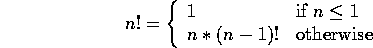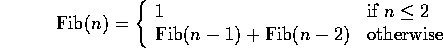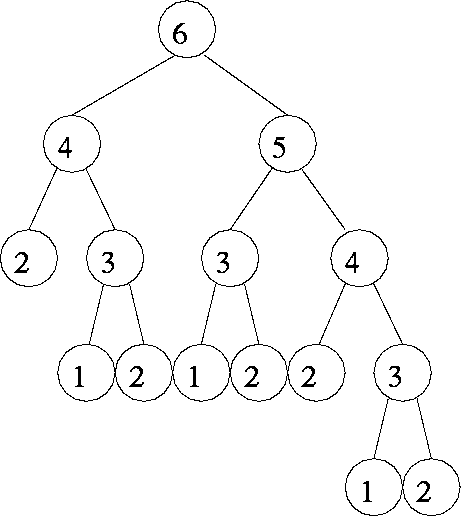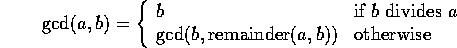# Introduction to Recursion

Tom Kelliher, CS18

Feb. 23, 1996

# Recursion

What is it?

A function that calls itself directly or indirectly to solve a smaller version of its task until a final call which does not require a self-call is a recursive function.

Divide and conquer approach

What do I need?

1. Decomposition into smaller problems of same type
2. Recursive calls must diminish problem size
3. Necessity of base case
4. Base case must be reached

## Binary Search Algorithm

Looking up a word in the dictionary?

• Sequential search
• Binary search

Algorithm:

```Search(dictionary)
{
if (Dictionary has only 1 page)
Sequentially search page for word
else
{
Open the dictionary to the middle page
Determine which half of the dictionary the word is in

if (The word is in the first half)
Search(first half of dictionary)   // ignore second half
else
Search(second half of dictionary   // ignore first half
}
}
```

Dictionary structure?

Compare to sequential search

## Factorial

Meet requirements?Consider the code fragment:

```main()
{
int i = 3;                 // 1
cout << f(i) << endl;      // 2
}

int f(int a1)
{
if (a1 <= 1)               // 3
return 1;               // 4
else                       // 5
return a1 * f(a1 - 1);  // 6
}
```

Non-recursive version:

```int fact(int n)
{
int i;
int prod = 1;

for (i = 1; i <= n; i++)
prod *= i;

return prod;
}
```

## The Fibonacci Sequence

Definition:Consider:

```int fib(int val)
{
if (val <= 2)
return 1;
else
return fib(val - 1) + fib(val - 2);
}
```

Call graph for fib(6):Non-recursive version:

```int fib(int val)
{
int current = 1;
int old = 1;
int older = 1;

val -=2;

while (val > 0)
{
current = old + older;
older = old;
old = current;
--val;
}

return current;
}
```

## Greatest Common Divisor

Definition:```int gcd(int a, int b)
{
int remainder = a % b;

if (remaider == 0)
return b;
else
return gcd(b, remainder);
}
```

## Fun with an Array

What does this do?

```int main()
{
int array = { 1, 2, 3, 4 };

mystery(array, 4);
}

void mystery(int array[], int size)
{
if (size > 0)
{
--size;
cout << array[size] << endl;
mystery(array, size);
}
}
```

```int mystery(int array[], int size)
{
--size;

if (size > 0)
return a[size] + mystery(array, size);
else
return a[size];
}
```

## Exercise

Write a recursive function which finds the maximum value stored in an int array.

Thomas P. Kelliher
Thu Feb 22 08:34:49 EST 1996
Tom Kelliher